• 使用 matplotlibpyplot模块绘制图形。看一个 绘制sin函数曲线的例子。 import matplotlib.pyplot as plt import numpy as np # 生成数据 x = np.arange(0, 6, 0.1) # 以0.1为单位，生成0到 6 的数据* y = np.sin...
• 使用 matplotlibpyplot模块绘制图形。看一个 绘制sin函数曲线的例子。 import matplotlib.pyplot as plt import numpy as np # 生成数据 x = np.arange(0, 6, 0.1) # 以0.1为单位，生成0到 6 的数据* y = np....
1.  绘制简单图形
使用 matplotlib 的pyplot模块绘制图形。看一个 绘制sin函数曲线的例子。
import matplotlib.pyplot as plt
import numpy as np

# 生成数据
x = np.arange(0, 6, 0.1) # 以0.1为单位，生成0到 6 的数据*
y = np.sin(x)

# 绘制图形
plt.plot(x,y)
plt.show()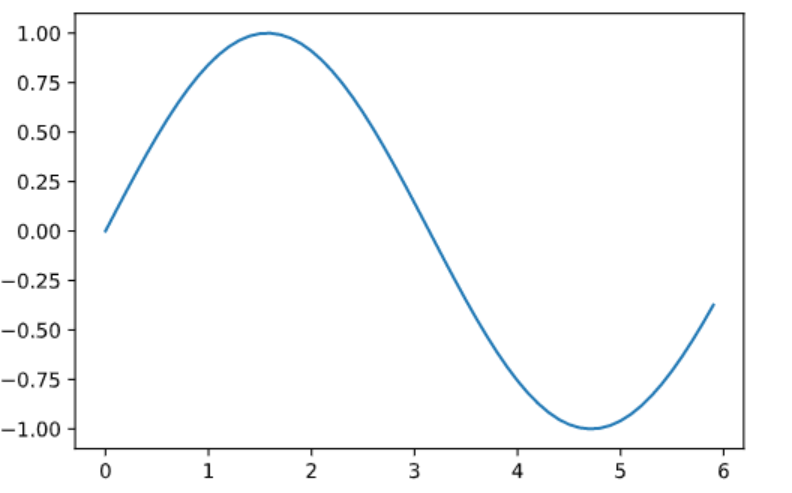这里使用NumPy的arange()方法生成了[0, 0.1, 0.2, … , 5.8, 5.9]的 数据，将其设为x。
对x的各个元素，应用NumPy的sin函数np.sin()，将x、 y的数据传给plt.plot方法，然后绘制图形。
最后，通过plt.show()显示图形。 运行上述代码后，就会显示如上图所示的图形。
2.  pyplot的功能
使用 pyplot的添加标题plt.title()、坐标轴标签名plt.xlabel()\ plt.ylabel()和图例plt.legend()。
import numpy as np
import matplotlib.pyplot as plt

# 生成数据
x = np.arange(0, 6, 0.1) # 以0.1为单位，生成0到6的数据
y1 = np.sin(x)
y2 = np.cos(x)

# 绘制图形
plt.plot(x, y1, label="sin")
plt.plot(x, y2, linestyle= "--", label="cos") # 用虚线绘制

plt.xlabel("x") # x轴标签
plt.ylabel("y") # y轴标签
plt.title('sin & cos') # 标题

plt.legend() #显示图例
plt.show()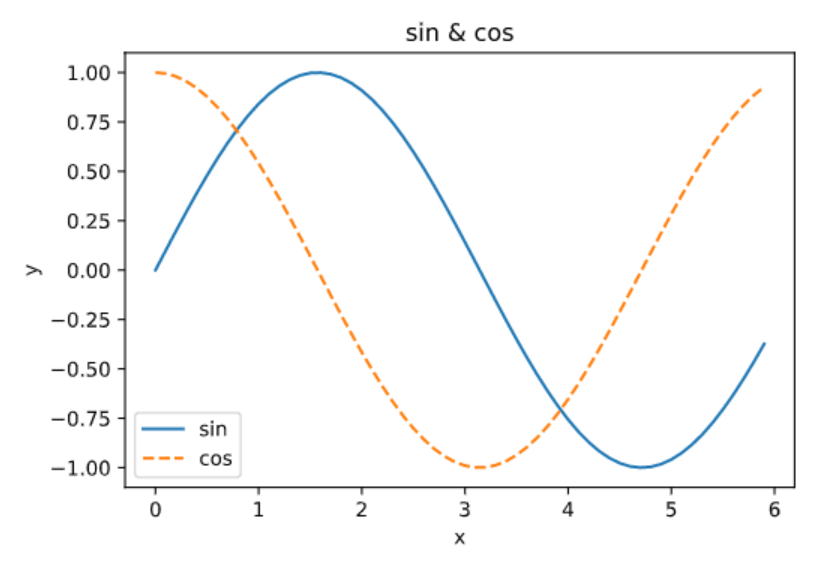3.  显示图像
pyplot中还提供了用于显示图像的方法imshow()。
使用 matplotlib.image模块的imread()方法读入图像。
import matplotlib.pyplot as plt

plt.imshow(img)

plt.show()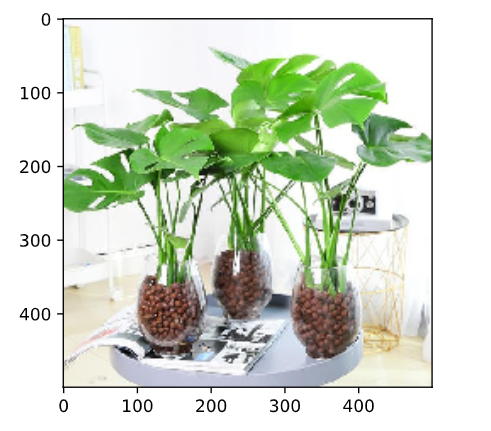展开全文python
• 模块matplotlib.pyplot提供了函数matplotlib.pyplot.subplot()用来向当前窗口内添加一个子窗口对象。该函数的语法格式为： matplotlib.pyplot.subplot(nrows,ncols,index) nrows为行数。 ncols为列数。 index为...


1.subplot函数

模块matplotlib.pyplot提供了函数matplotlib.pyplot.subplot()用来向当前窗口内添加一个子窗口对象。该函数的语法格式为：

matplotlib.pyplot.subplot(nrows,ncols,index)

nrows为行数。 ncols为列数。 index为窗口序号(序号是从1开始的）

import cv2
import matplotlib.pyplot as plt
equ=cv2.equalizeHist(img)
plt.figure("subplot")
plt.subplot(121),plt.hist(img.ravel(), 256)
plt.subplot(122),plt.hist(equ.ravel(), 256)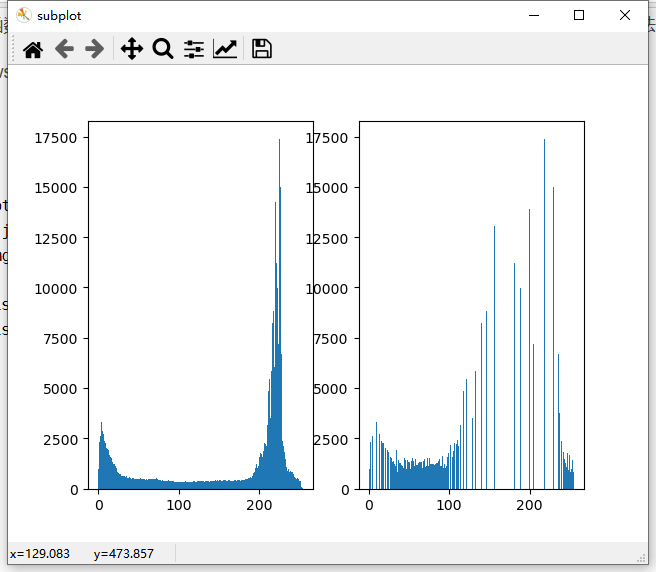2.matplotlib.pyplot.imshow()

matplotlib.pyplot.imshow(X，cmap=None)

式中：X为图像信息，可以是各种形式的数值，cmap表示色彩空间，该值是可选项，默认值为null，默认使用RGB(A)色彩空间。

import cv2
import matplotlib.pyplot as plt
imgRGB=cv2.cvtColor(img, cv2.COLOR_BGR2RGB)
plt.figure("xianshi")
plt.subplot(121)
plt.imshow(img),plt.axis('off')
plt.subplot(122)
plt.imshow(imgRGB),plt.axis('off')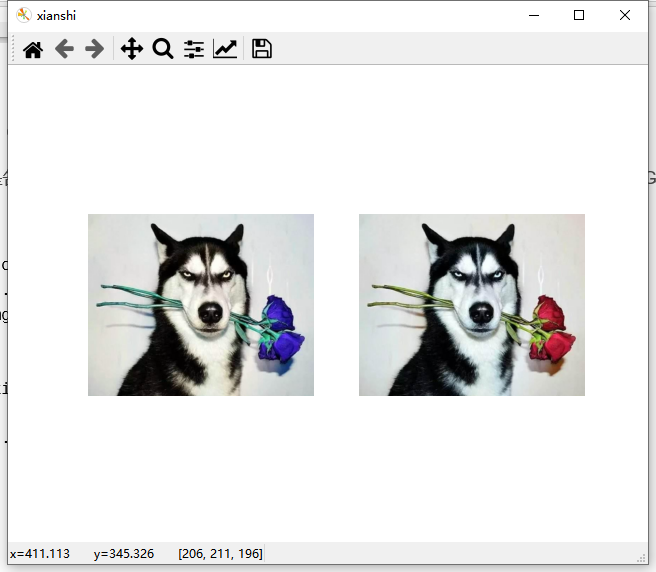import cv2
import matplotlib.pyplot as plt
g=cv2.cvtColor(o,cv2.COLOR_BGR2GRAY)
plt.figure("huidu")
plt.subplot(221)
plt.imshow(o),plt.axis('off')
plt.subplot(222)
plt.imshow(o,cmap=plt.cm.gray),plt.axis('off')
plt.subplot(223)
plt.imshow(g),plt.axis('off')
plt.subplot(224)
plt.imshow(g,cmap=plt.cm.gray),plt.axis('off')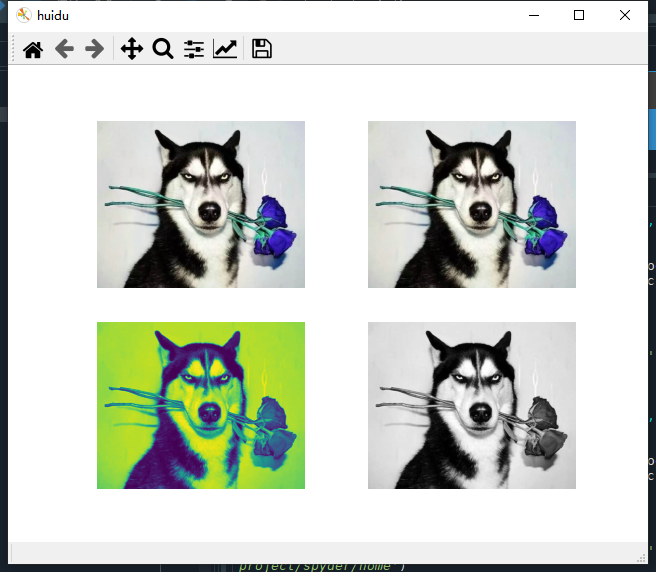● 左上角是原始图像。因为没有对读取的图像进行通道转换，直接将其作为彩色图像显示，所以显示结果中通道顺序是错乱的，图像没有正常显示。

● 右上角是我们尝试将彩色图像作为原始图像，将其色彩空间参数设置为“cmap=plt.cm.gray”，让彩色图像以灰度图像展示的结果，图像显示失败。

● 左下角是使用默认色彩空间参数，显示灰度图像的结果。这种情况下，灰度图像会采用默认的RGB模式显示。可以看到，图像没有正常显示。

● 右下角是将灰度图像作为原始图像，并将其色彩空间参数设置为“cmap=plt.cm.gray”而显示的灰度图像，图像显示正常。

import cv2
import matplotlib.pyplot as plt
g=cv2.cvtColor(o,cv2.COLOR_BGR2GRAY)
plt.figure('huidu')
plt.subplot(221)
plt.imshow(g,cmap=plt.cm.gray_r)
plt.subplot(222)
plt.imshow(g,cmap=plt.cm.gray)
plt.subplot(223)
plt.imshow(g,cmap='gray')
plt.subplot(224)
plt.imshow(g,cmap='gray_r')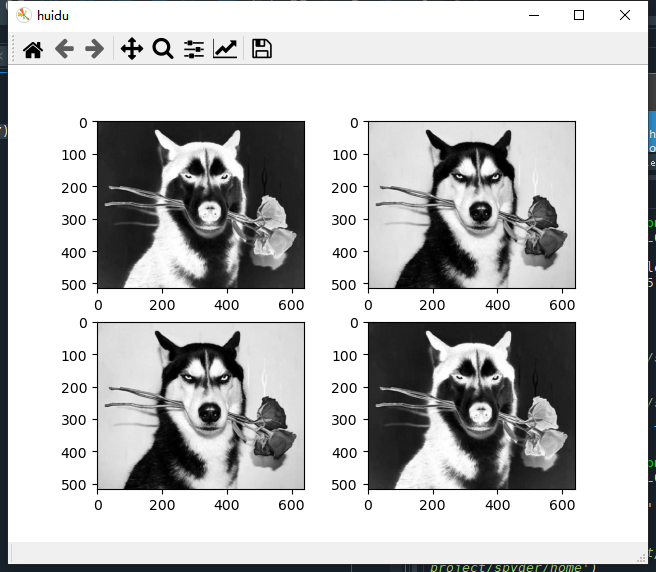色彩空间参数cmap的参数值“plt.cm.gray_r”及“gray_r”中的“r”是英文“reverse”的缩写，表示逆转的意思。


展开全文• 概述 xscale和yscale函数的作用都是设置坐标轴的缩放类型。其中 xscale函数作用是设置x轴的缩放类型。 yscale函数作用是设置y轴的缩放类型。 两者参数相同，仅功能...xscale函数的签名为：matplotlib.pyplot.xscale
概述
xscale和yscale函数的作用都是设置坐标轴的缩放类型。其中

xscale函数作用是设置x轴的缩放类型。
yscale函数作用是设置y轴的缩放类型。

两者参数相同，仅功能稍有不同。
xscale函数的签名为：matplotlib.pyplot.xscale(value, **kwargs)
yscale函数的签名为：matplotlib.pyplot.yscale(value, **kwargs)
xscale函数
xscale函数的签名为：matplotlib.pyplot.xscale(value, **kwargs)
默认的坐标轴缩放类型为："linear"
参数说明如下：

value：X轴的缩放类型。字符串，取值范围为{"linear", "log", "symlog", "logit", ...}或ScaleBase对象，自定义的缩放类型需要使用matplotlib.scale.register_scale注册。必备参数。
**kwargs：不同缩放类型接受的关键字参数不同。具体查看相关类：

matplotlib.scale.LinearScale
matplotlib.scale.LogScale
matplotlib.scale.SymmetricalLogScale
matplotlib.scale.LogitScale
matplotlib.scale.FuncScale

xscale函数的返回值为(locs, labels)元组。其中locs为X轴刻度位置列表，labels为X轴刻度标签列表
案例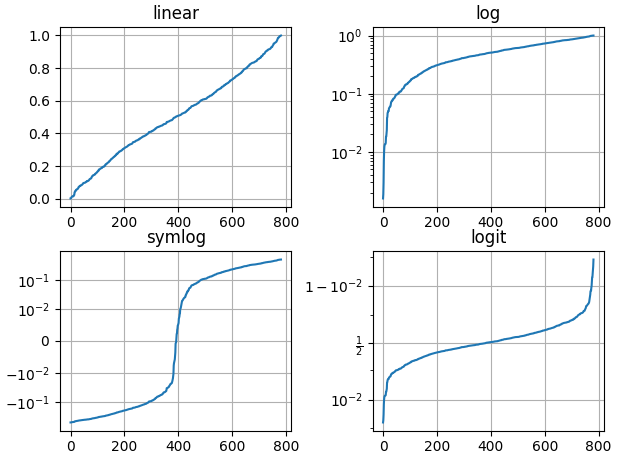import matplotlib.pyplot as plt
import numpy as np

np.random.seed(19880801)

# 构造数据，要求数据都在0-1之间，否则不能设置logit缩放类型
y = np.random.normal(loc=0.5, scale=0.4, size=1000)
y = y[(y > 0) & (y < 1)]
y.sort()
x = np.arange(len(y))

# linear，默认缩放类型
plt.subplot(221)
plt.plot(x, y)
plt.yscale('linear')
plt.title('linear')
plt.grid(True)

# log
plt.subplot(222)
plt.plot(x, y)
plt.yscale('log')
plt.title('log')
plt.grid(True)

# symmetric log
plt.subplot(223)
plt.plot(x, y - y.mean())
plt.yscale('symlog', linthresh=0.01)
plt.title('symlog')
plt.grid(True)

# logit
plt.subplot(224)
plt.plot(x, y)
plt.yscale('logit')
plt.title('logit')
plt.grid(True)

wspace=0.35)

plt.show()



展开全文XScale 缩放
• Matplotlib库提供了matplotlib.pyplot模块用于对图形的处理。对该模块的引用，统一使用如下格式要求：import matplotlib.pyplot as plt，后续在代码中发现plt别名，都是指向pyplot模块。 **1）、plot()，绘制基于坐...
绘制第一张图上
Matplotlib库提供了matplotlib.pyplot模块用于对图形的处理。对该模块的引用，统一使用如下格式要求：import matplotlib.pyplot as plt，后续在代码中发现plt别名，都是指向pyplot模块。
**1）、plot()，绘制基于坐标值（x,y）的点、线
函数plot(*args, fmt, data=None, **kwargs)，参数使用说明：
（1）*args，主要接受（x,y）坐标值，坐标可以是标量，x、y也可以是元组、列表、数组的值对。可以省略y值，则该函数默认坐标值x=y。
（2）fmt，字符串‘[color][marker][line]’，用于指定线的颜色、标记图标、线型。如’ro–’代表线是红色（r表示），坐标对应点上是o图标（o表示），线是虚线（–表示）。
（3）data，带有标记数据的对象。 如果给定，需要提供标签名称，并通过指定x,y坐标绘制。
（4）kwargs，用键值对形式指定线的一些属性，如linewidth=2用以指定线宽，color='green’代替fmt里的颜色指定方式， marker='o’指定图标，linestyle='dashed’指定线的风格，markersize=12指定图标大小。
   1、plot()，绘制基于坐标值（x,y）的点、线
（1）用标量坐标，绘制一个点

plt.plot(10,10,'o')    #为了直观直观查看(10,10)处的点，用o图标在该点做了标注
plt.show()   #显示绘图1、plot()，绘制基于坐标值（x,y）的点、线
（2）用数组坐标，绘制若干个点
import numpy as np
import matplotlib.pyplot as plt
x = np.array([0,10,5,5])   #这里x,y对应的坐标对包括了（0，5),(10,5),(5,0),(5,10)
y = np.array([5,5,0,10])
plt.plot(x,y,'o')   #这里必须用o图标，否则将产生线
plt.show()x = np.array([0,10,5,5])
y = np.array([5,5,0,10])
plt.plot(x,y)   #这里没有提供图标,就默认以线形式连接所有点
plt.show()用于绘图的plot()为点、线的颜色、图标标记、线型提供了丰富的功能，这里进一步介绍这些功能的用法。在plot()参数里颜色、图标、线型的设置有两种主要方法，一种是传递字符串参数，如’ro–’代表画红色的、o图标的、虚线的图形；另外一种采用键值对形式显式指定它们。
1、字符串设置
x = np.arange(0,5,0.02)
plt.plot(x,np.sin(2*np.pi*x),'go--')
plt.show()用于绘图的plot()为点、线的颜色、图标标记、线型提供了丰富的功能，这里进一步介绍这些功能的用法。在plot()参数里颜色、图标、线型的设置有两种主要方法，一种是传递字符串参数，如’ro–’代表画红色的、o图标的、虚线的图形；另外一种采用键值对形式显式指定它们。
2、键值对设置
x = np.arange(0,5,0.02)
plt.plot(x,np.sin(2*np.pi*x),color='r',marker='v',linestyle='-')  #r红色,v下三角图标,-实线
plt.show()展开全文python 可视化
• pyplot模块中，figure函数用于创建新的图形，或激活已存在的图形。 函数的签名为matplotlib.pyplot.figure(num=None, figsize=None, dpi=None, facecolor=None, edgecolor=None, frameon=True, FigureClass=<...figure 标识符 自定义
• pause函数的签名为matplotlib.pyplot.pause(interval)。参数interval表示秒数，类型为整数。 如果当前有活动的图形，在pause函数运行前，图形将会更新并显示，在等待期间事件循环会一直运行，只到暂停时间interval后...pause 事件循环 阻塞
• PyCharm导入matplotlib.pyplot模块报错解决办法 今天可是被这个问题折磨惨了。 明明已经检查了matplotlib也已经安装好了 导入pyplot模块就是报错 这里我就直接说自己的问题了 我寻思着，这不刚开始学习matplotlib吗...python
• fill函数的签名为：matplotlib.pyplot.fill(*args, data=None, **kwargs) 参数说明如下： *args：根据x,y位置确定结点绘制多边形，可添加一个可选的颜色标记。数据结构为x, y, [color]序列，支持多组x, y, [color]...fill 填充 多边形
• 函数的签名为matplotlib.pyplot.errorbar(x, y, yerr=None, xerr=None, fmt='', ecolor=None, elinewidth=None, capsize=None, barsabove=False, lolims=False, uplims=False, xlolims=False, xuplims=False, ererrorbar plot
• 当前有效matplotlib版本为：3.4.1。...函数的定义签名为matplotlib.pyplot.savefig(*args, **kwargs)。 函数的调用签名为：savefig(fname, dpi=None, facecolor='w', edgecolor='w', orientation='portrait'savefig 格式
• 函数的定义签名为：matplotlib.pyplot.subplots(nrows=1, ncols=1, *, sharex=False, sharey=False, squeeze=True, subplot_kw=None, gridspec_kw=None, **fig_kw) 函数的参数为： nrows, ncols : 子图网格的行/列...subplot squeeze
• 概述 subplot()函数向当前图像（figure）...函数的定义签名为：matplotlib.pyplot.subplot(*args, **kwargs) 函数的调用签名为： subplot(nrows, ncols, index, **kwargs) subplot(pos, **kwargs) subplot(**kwargs) susubplot
• python中调用matplotlib.pyplot模块不显示图片，如下： 加上plt.show()即可显示
• -- coding: utf-8 -- from future import unicode_literals ...import matplotlib.pyplot as mp import matplotlib.animation as ma mp.figure(“Signal”)#创建窗口对象 mp.xlabel(‘Time’)#设置x...Python
• 函数的签名为matplotlib.pyplot.table(cellText=None, cellColours=None, cellLoc='right', colWidths=None, rowLabels=None, rowColours=None, rowLoc='left', colLabels=None, colColours=None, colLoc='center', ...表格 table
• from matplotlib import pyplot as plt x=range(2,13,2) y=[9,32,14,17,23,7] #setting size of pic plt.figure(figsize=(20,8),dpi=80) #painting plt.plot(x,y) #set the pos of x the level plt.xticks(range(7,2...python 数据可视化 可视化
• 概述 xsticks和ysticks函数的作用都是获取或设置坐标轴的刻度及标签。其中 xsticks函数作用是获取或设置x坐标轴的刻度及标签。...ysticks函数的签名为：matplotlib.pyplot.yticks(ticks=None, labels=None,标签 ticks
• 函数的签名为matplotlib.pyplot.findobj(o=None, match=None, include_self=True)。 函数的参数为： o：容器对象，即需要查找子对象的对象。默认值为None，即当前图形对象。 match：匹配规则。默认值为None。取值...findobj artist 对象
• 1. 绘制简单图形使用 matplotlibpyplot模块绘制图形。看一个 绘制sin函数曲线的例子。import matplotlib.pyplot as pltimport numpy as np# 生成数据x = np.arange(0, 6, 0.1) # 以0.1为单位，生成0到 6 的数据*y...
• matplotlibpyplot模块plot函数基础一（函数功能、xy参数基本取值，多组数据）简单说明了plot函数绘制线条的基本功能。 plot函数的基本调用签名为plot([x], y, [fmt], *, data=None, **kwargs) 那么线条的外观该...plot fmt
• pyplot模块中可以使用xlabel()和ylabel()函数设置x轴y轴的标签。这两个函数的使用方法非常相似。 使用xlabel()设置x轴标签 函数签名为matplotlib.pyplot.xlabel(xlabel, fontdict=None, labelpad=None, *, loc=...坐标轴标签 xlabel
• 函数签名为matplotlib.pyplot.title(label, fontdict=None, loc=None, pad=None, *, y=None, **kwargs) 参数作用及取值如下： label：类型为字符串，即标题文本。 fontdict：类型为字典，控制文本标题 title
• 函数的签名为matplotlib.pyplot.arrow(x, y, dx, dy, **kwargs)。 函数的参数为： x, y：箭头尾部的坐标。类型为浮点数。必备参数。 dx, dy：箭头在xy方向的长度。类型为浮点数。必备参数。 width：箭头尾部的宽度...arrow
• 当前有效matplotlib版本为：3.4.1。 概述 axes()函数功能与subplot()函数极其相似。...函数的定义签名为：matplotlib.pyplot.axes(arg=None, **kwargs) 函数的调用签名为： # 在当前图像中添加一个铺满的子图 plt.axaxes
• pie()的函数签名为matplotlib.pyplot.pie(x, explode=None, labels=None, colors=None, autopct=None, pctdistance=0.6, shadow=False, labeldistance=1.1, startangle=0, radius=1, counterclock=True, wedgeprops=...饼图
• text函数的签名为：matplotlib.pyplot.text(x, y, s, fontdict=None, **kwargs) 参数说明如下： x，y：放置文本的坐标。浮点数。必备参数。 s：文本。字符串。必备参数。 fontdict：字体属性字典，用于覆盖默认文本...python text annotate 注解
• 1、导入numpy,matplotlib.pyplot模块，并将其别名 import numpy as np import matplotlib.pyplot as plt 2、读取图片 :cat = plt.imread(‘cat.jpg’) # 输出结果： array([[[231, 186, 131], [232, 187, 132]...python
• matplotlib.pyplot模块中的函数axis()只能用于设置坐标轴的状态嘛？有问必答 python...